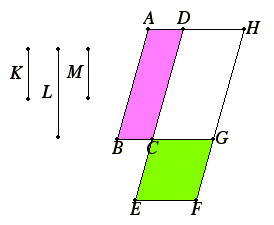# Proposition 23

Equiangular parallelograms have to one another the ratio compounded of the ratios of their sides.

Let AC and CF be equiangular parallelograms having the angle BCD equal to the angle ECG.

I say that the parallelogram AC has to the parallelogram CF the ratio compounded of the ratios of the sides.

I.14

Let them be placed so that BC is in a straight line with CG. Then DC is also in a straight line with CE.Complete the parallelogram DG. Set out a straight line K, and make it so that BC is to CG as K is to L, and DC is to CE as L is to M.

Then the ratios of K to L and of L to M are the same as the ratios of the sides, namely of BC to CG and of DC to CE.

But the ratio of K to M is compounded of the ratio of K to L and of that of L to M, so that K has also to M the ratio compounded of the ratios of the sides.

Now since BC is to CG as the parallelogram AC is to the parallelogram CH, and BC is to CG as K is to L, therefore K is to L as AC is to CH.

Again, since DC is to CE as the parallelogram CH is to CF, and DC is to CE as L is to M, therefore L is to M as the parallelogram CH is to the parallelogram CF.

V.22

Since then it was proved that K is to L as the parallelogram AC is to the parallelogram CH, and L is to M as the parallelogram CH is to the parallelogram CF, therefore, ex aequali K is to M as AC is to the parallelogram CF.

But K has to M the ratio compounded of the ratios of the sides, therefore AC also has to CF the ratio compounded of the ratios of the sides.

Therefore, equiangular parallelograms have to one another the ratio compounded of the ratios of their sides.

Q.E.D.

## Guide

This proposition is a generalization of the basic formula for the area of a rectangle, that is, the area of a rectangle is the product of its length and width. Such a formula depends on predetermined units of length and area so that the unit area is the area of a square whose sides have length equal to the unit length. Euclid and other Greek mathematicians did not use predetermined units of length or area, so they expressed this formula as a proportion. We would state that proportion as saying the ratio of the area of a given rectangle to the area of a given square is the product of the ratios of the lengths of the sides of the rectangle to the length of a side of the square. Of course, Euclid would say that without using the words area and length as follows: the ratio of the a given rectangle to a given square is the product of the ratios of the sides of the rectangle to a side of the square.

Note that his terminology for a product of ratios involves “compounding the ratios.” A natural generalization of the ratio of a rectangle to a square is the ratio of a rectangle to a rectangle. A broader generalization is the ratio of one parallelogram to another parallelogram having the same angles. That gives the generalization as stated in this proposition.

#### Areas of rectangles and parallelograms

These areas have been treated earlier in the Elements. Back in Book I and II the basic concept was “quadrature,” that is, finding a square or other shaped figure of the same area as the given rectangle or parallelogram. That began with Proposition I.35 which said two triangles on the same base and with the same height are equal, and ended with I.14 which constructed a square equal to a given rectangle.

Early in this book was the proposition VI.1 generalizing I.35 which said that parallelograms with the same height are proportional to their bases. Finally, in this proposition we have the full statement about areas of rectangles and parallelograms.

#### Analogous statements in other books

Proposition VIII.5 states that plane numbers have to one another the ratio compounded of the ratios of their sides. That proposition is probably a much older version that may go back to the Pythagoreans when “all was number.” The discovery of incommensurable lines showed there were serious limitations to that version of the proposition.

In Book XI there are analogous statements for volumes of parallelepipeds. For instance, Proposition XI.33 states that similar parallelepipeds are to one another in the triplicate ratio of their corresponding sides. That statement for parallelepipeds is analogous to this one for parallelograms.

#### Use of this theorem

Although this is a basic proposition on areas, it is actually not used in the rest of the Elements.